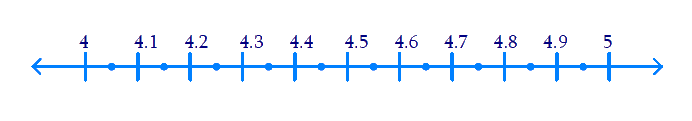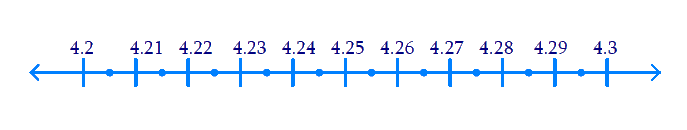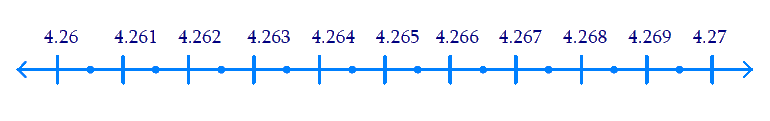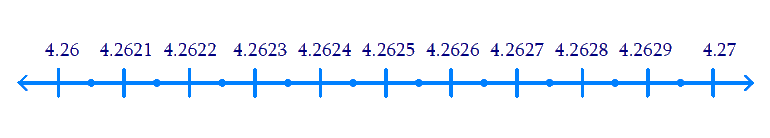# Ex.1.4 Q2 Number System Solution - NCERT Maths Class 9

Go back to  'Ex.1.4'

## Question

Visualize \begin{align}4 . \overline{26}\end{align} on the number line, up to $$4$$ decimal places.

Video Solution
Number Systems
Ex 1.4 | Question 2

## Text Solution

Steps:

\begin{align}4 . \overline{26}=4.2626\dots\end{align}

(i) $$4.2$$ lies between $$4$$ &$$5$$. Observe the number line given below(ii) $$4.26$$ lies between $$4.2$$ & $$4.3$$, shown below on the number line.(iii) $$4.262$$ lies between $$4.26$$ & $$4.27$$ as shown below.(iv) $$4.2626$$ lies between $$4.262$$ & $$4.263$$ as shown belowHence by the successive magnification method required number is obtained in the number line.

Video Solution
Number Systems
Ex 1.4 | Question 2

Learn from the best math teachers and top your exams

• Live one on one classroom and doubt clearing
• Practice worksheets in and after class for conceptual clarity
• Personalized curriculum to keep up with school